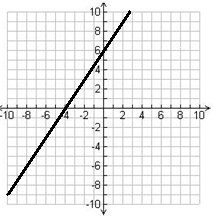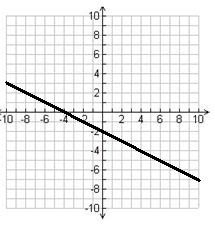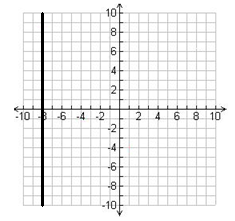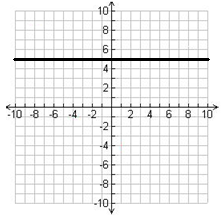Graphing linear functions using a single point and slope

Graphing linear functions using a single point and slope

If we are given a single point and the slope of a straight line, we will be able to graph it. By the same token, we can determine the slope and intercepts if we are given from its graph.

Lessons

• 1.
Graph the following line that passes through the given point and the slope
a)
$(-3, 2) ;$m =${2 \over 3}$

b)
$(4, -2);$m = -${3 \over 5}$

c)
$(1, 2);$m = undefined

d)
$(2, 5);$m = 0

e)
x int = 2; m = -1

f)
y int = -3; m = ${3 \over 2}$

• 2.
Find the slope, x and y intercepts of each line.
a)b)c)d)